Implementation of a selection of gradient palettes available in cpt-city.

The following scales and palettes are provided:

• scale_*_hypso_d(): For discrete values.

• scale_*_hypso_c(): For continuous values.

• scale_*_hypso_b(): For binning continuous values.

• hypso.colors(): A gradient color palette. See also grDevices::terrain.colors() for details.

An additional set of scales is provided. These scales can act as hypsometric (or bathymetric) tints.

• scale_*_hypso_tint_d(): For discrete values.

• scale_*_hypso_tint_c(): For continuous values.

• scale_*_hypso_tint_b(): For binning continuous values.

• hypso.colors2(): A gradient color palette. See also grDevices::terrain.colors() for details.

See Details.

Additional parameters ... would be passed on to:

• Discrete values: ggplot2::discrete_scale()

• Continuous values: ggplot2::continuous_scale()

• Binned continuous values: ggplot2::binned_scale().

Note that tidyterra just documents a selection of these additional parameters, check the previous links to see the full range of parameters accepted by these scales.

## Usage

scale_fill_hypso_d(
palette = "etopo1_hypso",
...,
alpha = 1,
direction = 1,
na.translate = FALSE,
drop = TRUE
)

scale_colour_hypso_d(
palette = "etopo1_hypso",
...,
alpha = 1,
direction = 1,
na.translate = FALSE,
drop = TRUE
)

scale_fill_hypso_c(
palette = "etopo1_hypso",
...,
alpha = 1,
direction = 1,
na.value = "transparent",
guide = "colourbar"
)

scale_colour_hypso_c(
palette = "etopo1_hypso",
...,
alpha = 1,
direction = 1,
na.value = "transparent",
guide = "colourbar"
)

scale_fill_hypso_b(
palette = "etopo1_hypso",
...,
alpha = 1,
direction = 1,
na.value = "transparent",
guide = "coloursteps"
)

scale_colour_hypso_b(
palette = "etopo1_hypso",
...,
alpha = 1,
direction = 1,
na.value = "transparent",
guide = "coloursteps"
)

hypso.colors(n, palette = "etopo1_hypso", alpha = 1, rev = FALSE)

scale_fill_hypso_tint_d(
palette = "etopo1_hypso",
...,
alpha = 1,
direction = 1,
na.translate = FALSE,
drop = TRUE
)

scale_colour_hypso_tint_d(
palette = "etopo1_hypso",
...,
alpha = 1,
direction = 1,
na.translate = FALSE,
drop = TRUE
)

scale_fill_hypso_tint_c(
palette = "etopo1_hypso",
...,
alpha = 1,
direction = 1,
values = NULL,
limits = NULL,
na.value = "transparent",
guide = "colourbar"
)

scale_colour_hypso_tint_c(
palette = "etopo1_hypso",
...,
alpha = 1,
direction = 1,
values = NULL,
limits = NULL,
na.value = "transparent",
guide = "colourbar"
)

scale_fill_hypso_tint_b(
palette = "etopo1_hypso",
...,
alpha = 1,
direction = 1,
values = NULL,
limits = NULL,
na.value = "transparent",
guide = "coloursteps"
)

scale_colour_hypso_tint_b(
palette = "etopo1_hypso",
...,
alpha = 1,
direction = 1,
values = NULL,
limits = NULL,
na.value = "transparent",
guide = "coloursteps"
)

hypso.colors2(n, palette = "etopo1_hypso", alpha = 1, rev = FALSE)

## Arguments

palette

A valid palette name. The name is matched to the list of available palettes, ignoring upper vs. lower case. See hypsometric_tints_db for more info. Values available are: "arctic", "arctic_bathy", "arctic_hypso", "c3t1", "colombia", "colombia_bathy", "colombia_hypso", "dem_poster", "dem_print", "dem_screen", "etopo1", "etopo1_bathy", "etopo1_hypso", "gmt_globe", "gmt_globe_bathy", "gmt_globe_hypso", "meyers", "meyers_bathy", "meyers_hypso", "moon", "moon_bathy", "moon_hypso", "nordisk-familjebok", "nordisk-familjebok_bathy", "nordisk-familjebok_hypso", "pakistan", "spain", "usgs-gswa2", "utah_1", "wiki-2.0", "wiki-2.0_bathy", "wiki-2.0_hypso", "wiki-schwarzwald-cont".

...

Arguments passed on to ggplot2::discrete_scale, ggplot2::continuous_scale, ggplot2::binned_scale

breaks

One of:

• NULL for no breaks

• waiver() for the default breaks (the scale limits)

• A character vector of breaks

• A function that takes the limits as input and returns breaks as output. Also accepts rlang lambda function notation.

labels

One of:

• NULL for no labels

• waiver() for the default labels computed by the transformation object

• A character vector giving labels (must be same length as breaks)

• An expression vector (must be the same length as breaks). See ?plotmath for details.

• A function that takes the breaks as input and returns labels as output. Also accepts rlang lambda function notation.

expand

For position scales, a vector of range expansion constants used to add some padding around the data to ensure that they are placed some distance away from the axes. Use the convenience function expansion() to generate the values for the expand argument. The defaults are to expand the scale by 5% on each side for continuous variables, and by 0.6 units on each side for discrete variables.

minor_breaks

One of:

• NULL for no minor breaks

• waiver() for the default breaks (one minor break between each major break)

• A numeric vector of positions

• A function that given the limits returns a vector of minor breaks. Also accepts rlang lambda function notation.

n.breaks

An integer guiding the number of major breaks. The algorithm may choose a slightly different number to ensure nice break labels. Will only have an effect if breaks = waiver(). Use NULL to use the default number of breaks given by the transformation.

nice.breaks

Logical. Should breaks be attempted placed at nice values instead of exactly evenly spaced between the limits. If TRUE (default) the scale will ask the transformation object to create breaks, and this may result in a different number of breaks than requested. Ignored if breaks are given explicitly.

alpha

The alpha transparency, a number in [0,1], see argument alpha in hsv.

direction

Sets the order of colors in the scale. If 1, the default, colors are ordered from darkest to lightest. If -1, the order of colors is reversed.

na.translate

Should NA values be removed from the legend? Default is TRUE.

drop

Should unused factor levels be omitted from the scale? The default (TRUE) removes unused factors.

na.value

Missing values will be replaced with this value. By default, tidyterra uses na.value = "transparent" so cells with NA are not filled. See also #120.

guide

A function used to create a guide or its name. See guides() for more information.

n

the number of colors ($$\ge 1$$) to be in the palette.

rev

logical indicating whether the ordering of the colors should be reversed.

values

if colours should not be evenly positioned along the gradient this vector gives the position (between 0 and 1) for each colour in the colours vector. See rescale() for a convenience function to map an arbitrary range to between 0 and 1.

limits

One of:

• NULL to use the default scale range

• A numeric vector of length two providing limits of the scale. Use NA to refer to the existing minimum or maximum

• A function that accepts the existing (automatic) limits and returns new limits. Also accepts rlang lambda function notation. Note that setting limits on positional scales will remove data outside of the limits. If the purpose is to zoom, use the limit argument in the coordinate system (see coord_cartesian()).

## Value

The corresponding ggplot2 layer with the values applied to the fill/colour aesthetics.

## Details

On scale_*_hypso_tint_* palettes, the position of the gradients and the limits of the palette are redefined. Instead of treating the color palette as a continuous gradient, they are rescaled to act as a hypsometric tint. A rough description of these tints are:

• Blue colors: Negative values.

• Green colors: 0 to 1.000 values.

• Browns: 1000 to 4.000 values.

• Whites: Values higher than 4.000.

The following orientation would vary depending on the palette definition (see hypsometric_tints_db for an example on how this could be achieved).

Note that the setup of the palette may not be always suitable for your specific data. For example, raster of small parts of the globe (and with a limited range of elevations) may not be well represented. As an example, a raster with a range of values on [100, 200] would appear almost as an uniform color.

This could be adjusted using the limits/values provided by ggplot2.

hypso.colors2() provides a gradient color palette where the distance between colors is different depending of the type of color. In contrast, hypso.colors() provides an uniform gradient across colors. See Examples.

hypsometric_tints_db, terra::plot(), terra::minmax(), ggplot2::scale_fill_viridis_c()

See also ggplot2 docs on additional ... parameters:

• scale_*_terrain_d(): For discrete values.

• scale_*_terrain_c(): For continuous values.

• scale_*_terrain_b(): For binning continuous values.

Other gradient scales and palettes for hypsometry: scale_color_coltab(), scale_cross_blended, scale_terrain, scale_whitebox

## Examples

# \donttest{
filepath <- system.file("extdata/volcano2.tif", package = "tidyterra")

library(terra)
volcano2_rast <- rast(filepath)

# Palette
plot(volcano2_rast, col = hypso.colors(100, palette = "wiki-2.0_hypso"))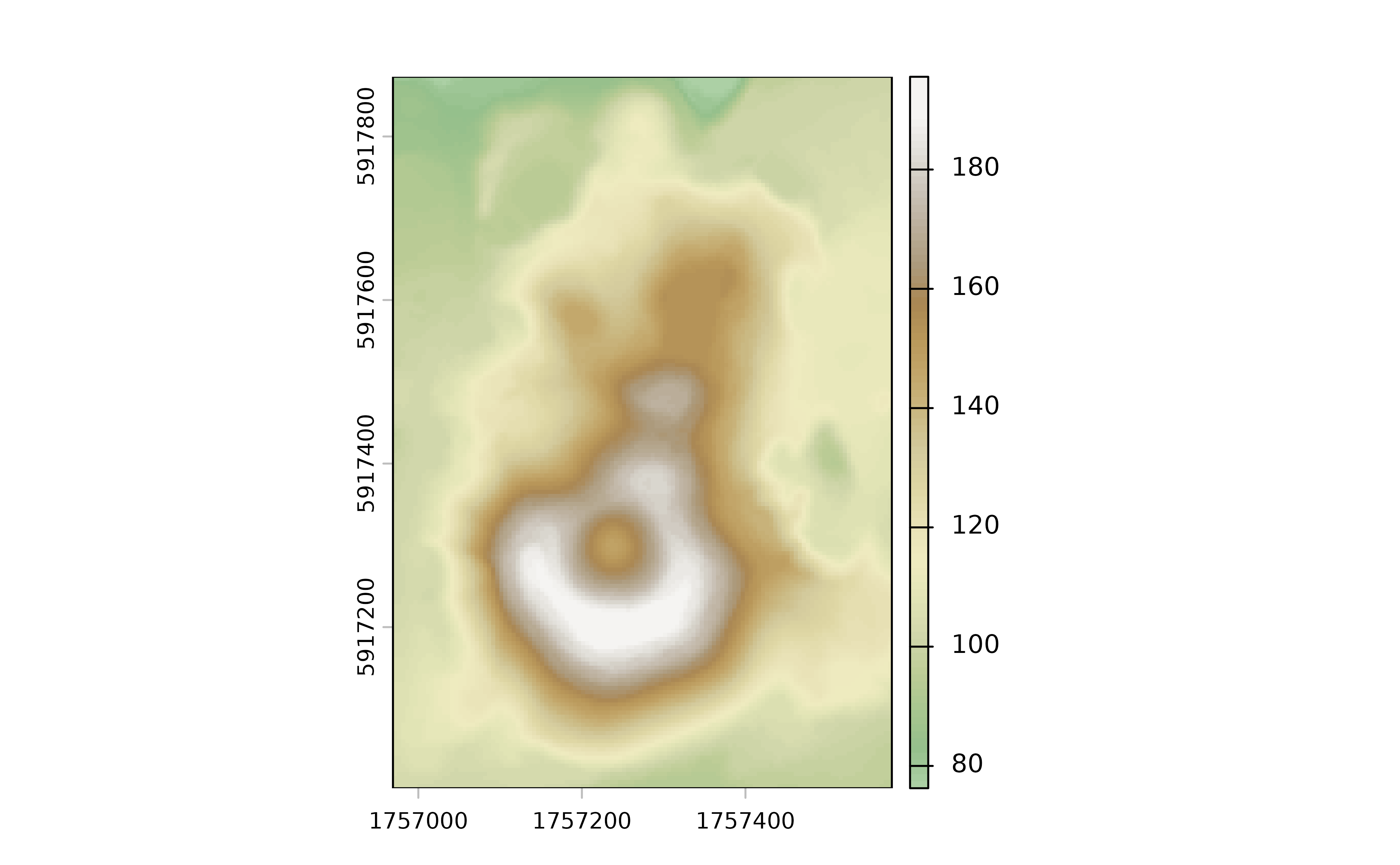# Palette with uneven colors
plot(volcano2_rast, col = hypso.colors2(100, palette = "wiki-2.0_hypso"))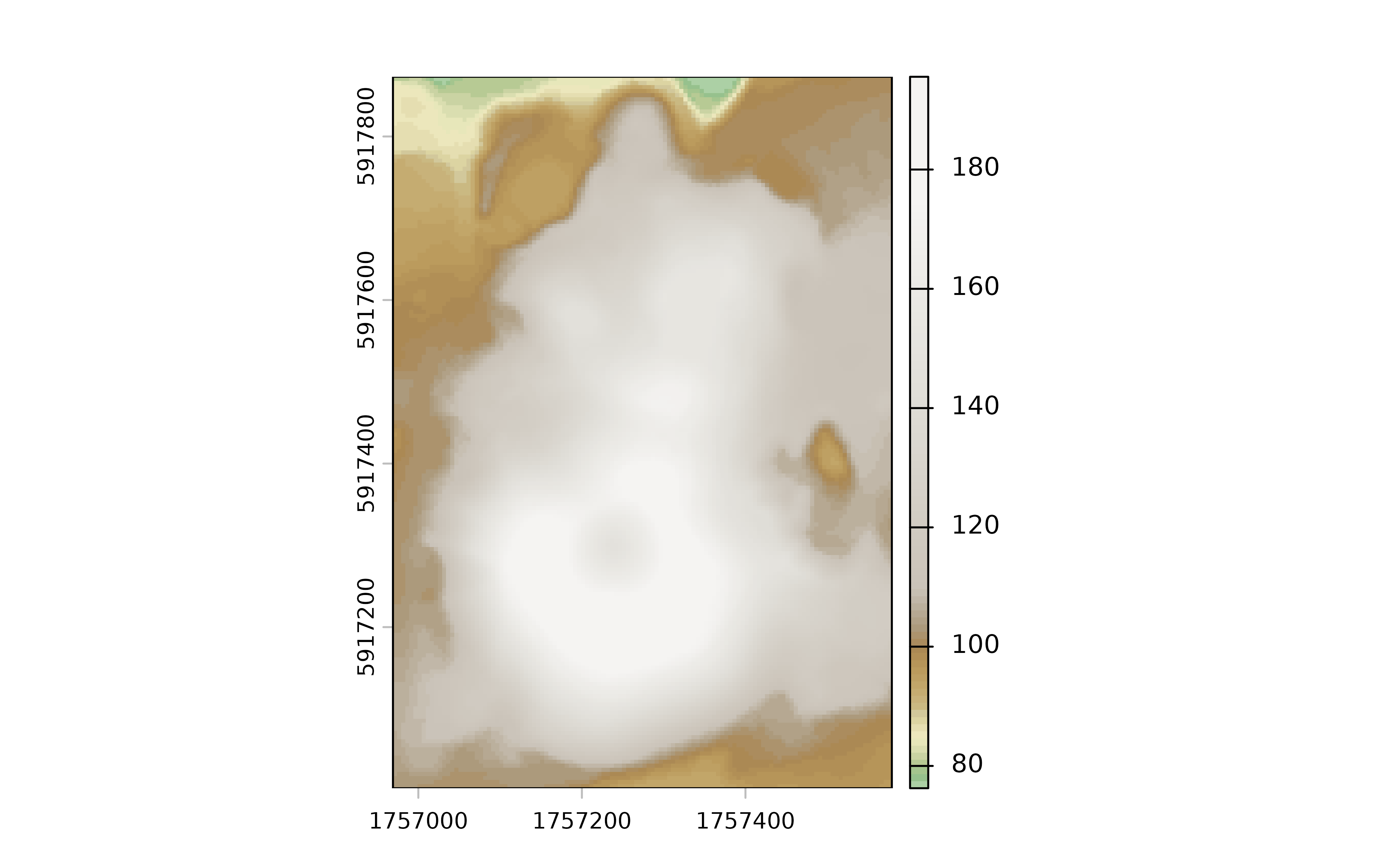library(ggplot2)
ggplot() +
geom_spatraster(data = volcano2_rast) +
scale_fill_hypso_c(palette = "colombia_hypso")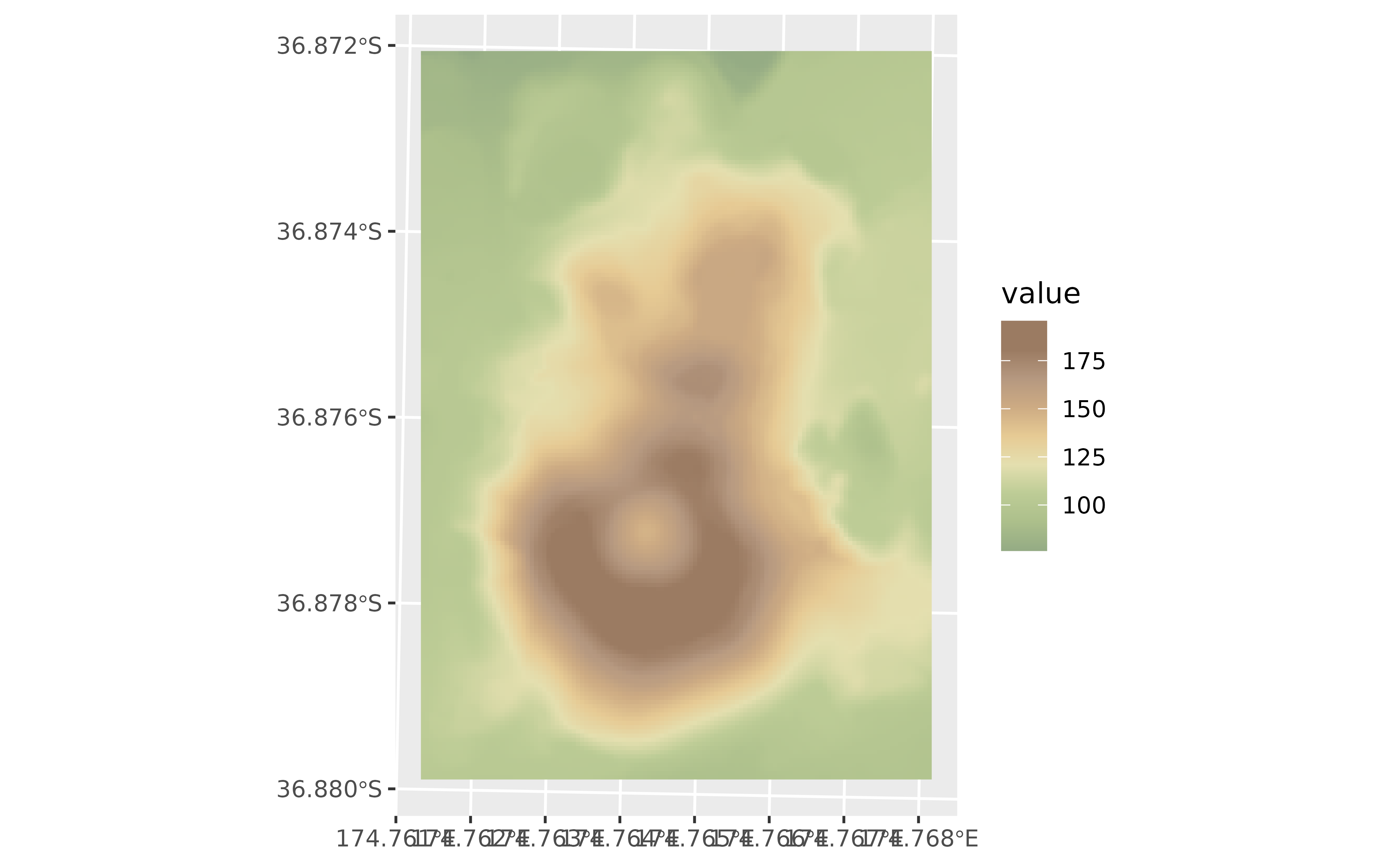# Use hypsometric  tint version...
ggplot() +
geom_spatraster(data = volcano2_rast) +
scale_fill_hypso_tint_c(palette = "colombia_hypso")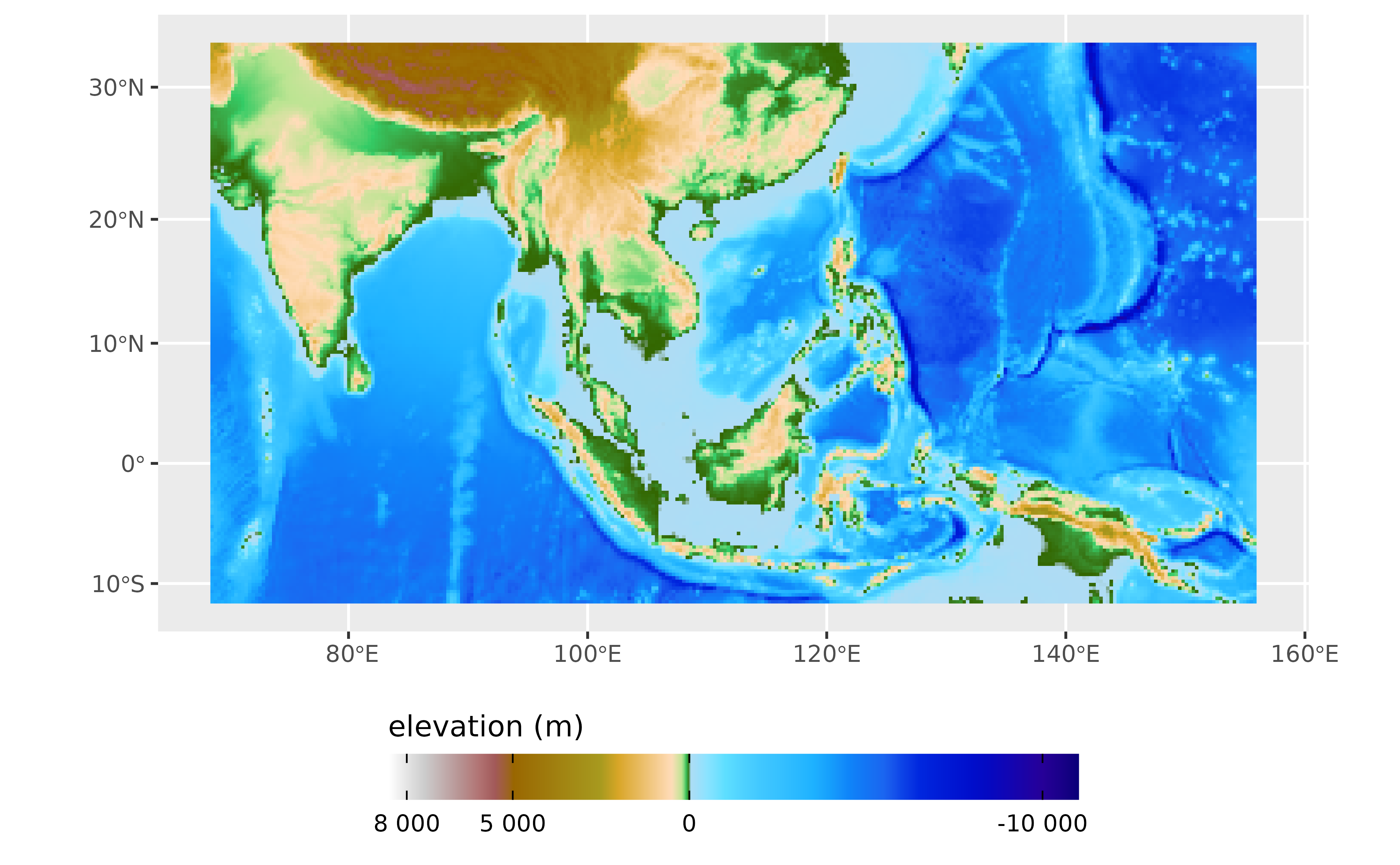# ...but not suitable for the range of the raster: adjust
my_lims <- minmax(volcano2_rast) %>% as.integer() + c(-2, 2)

ggplot() +
geom_spatraster(data = volcano2_rast) +
scale_fill_hypso_tint_c(
palette = "colombia_hypso",
limits = my_lims
)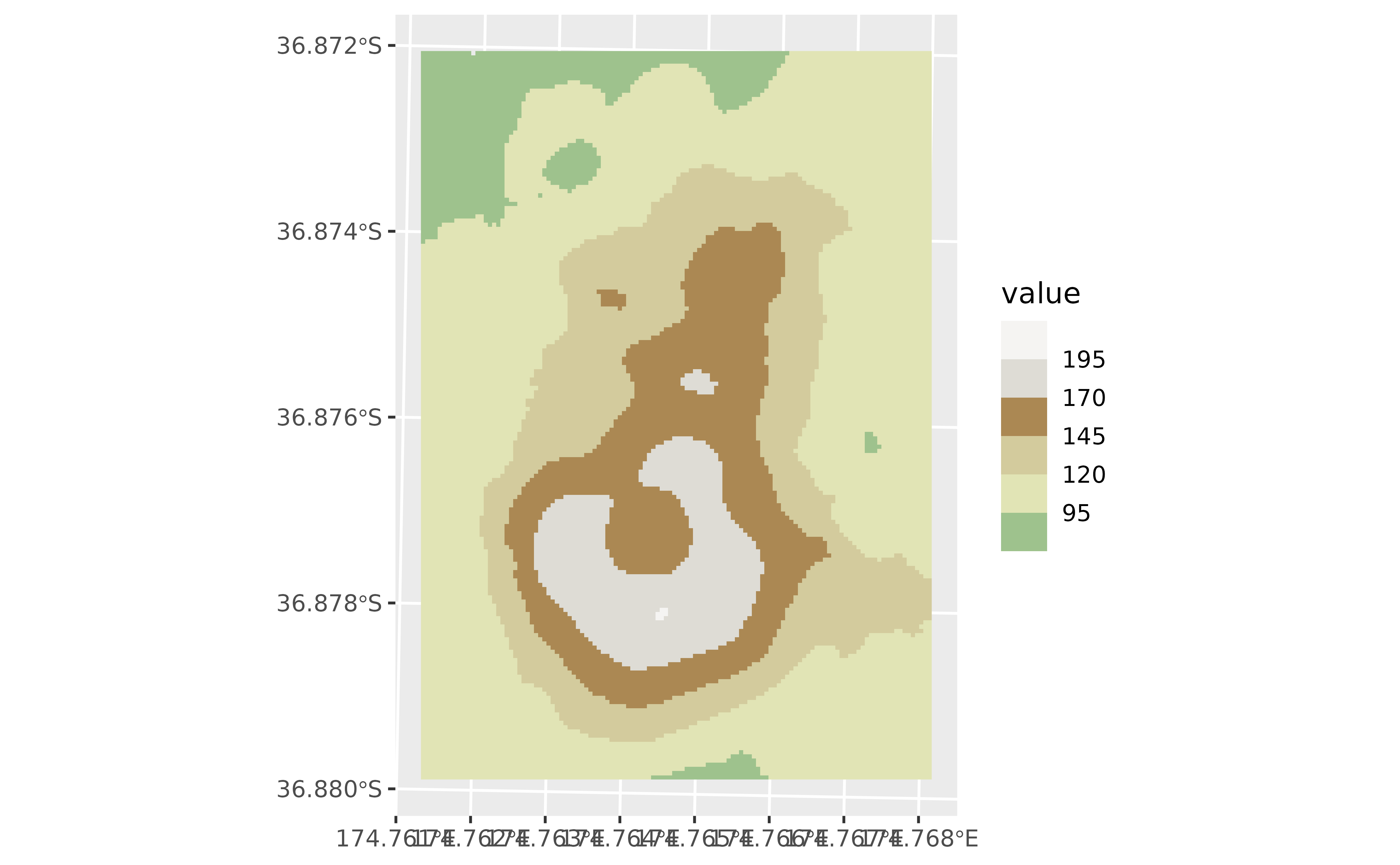# Full map with true tints

f_asia <- system.file("extdata/asia.tif", package = "tidyterra")
asia <- rast(f_asia)

ggplot() +
geom_spatraster(data = asia) +
scale_fill_hypso_tint_c(
palette = "etopo1",
labels = scales::label_number(),
breaks = c(-10000, 0, 5000, 8000),
guide = guide_colorbar(
direction = "horizontal",
title.position = "top",
barwidth = 25
)
) +
labs(fill = "elevation (m)") +
theme_minimal() +
theme(legend.position = "bottom")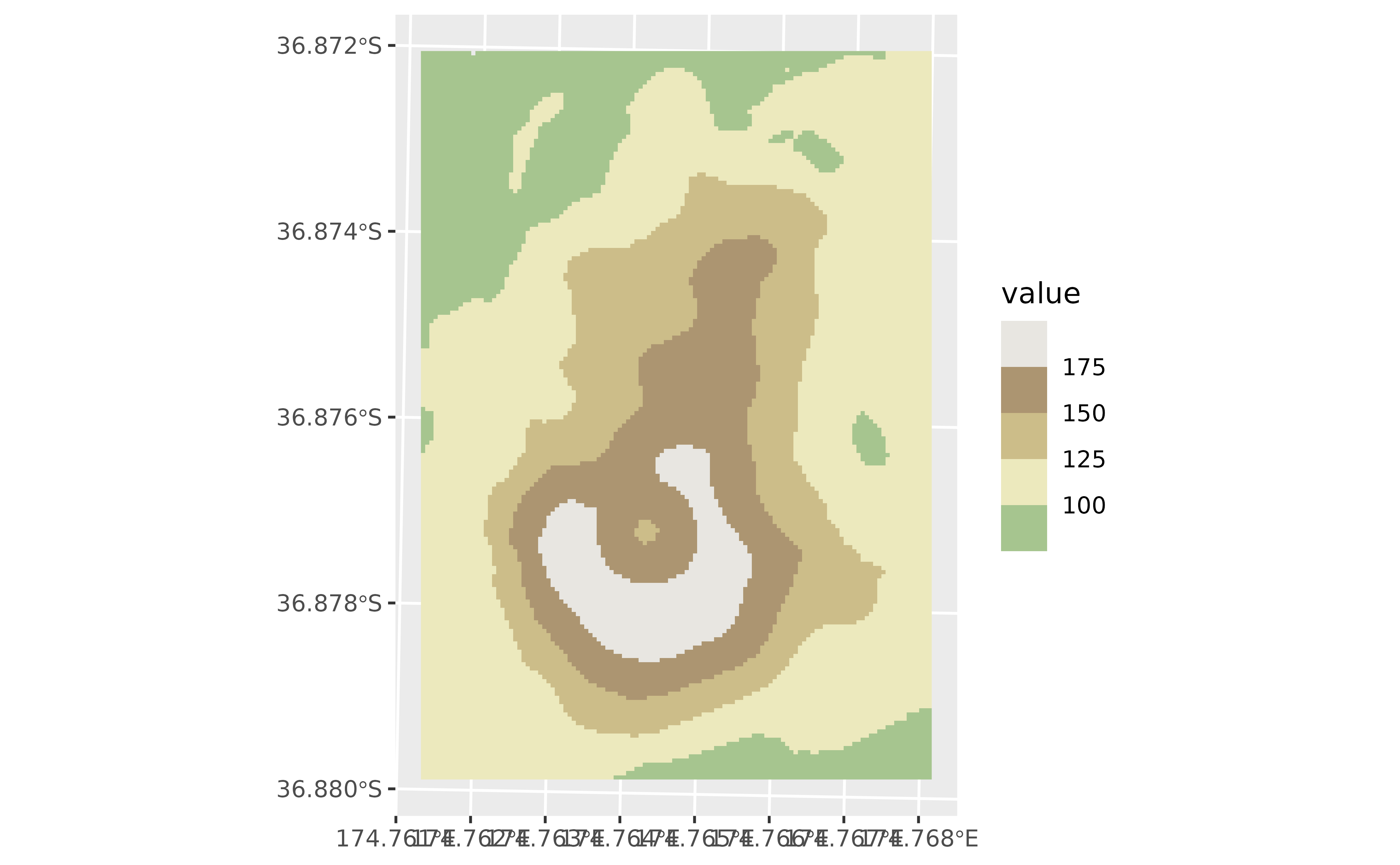# Binned
ggplot() +
geom_spatraster(data = volcano2_rast) +
scale_fill_hypso_b(breaks = seq(70, 200, 25), palette = "wiki-2.0_hypso")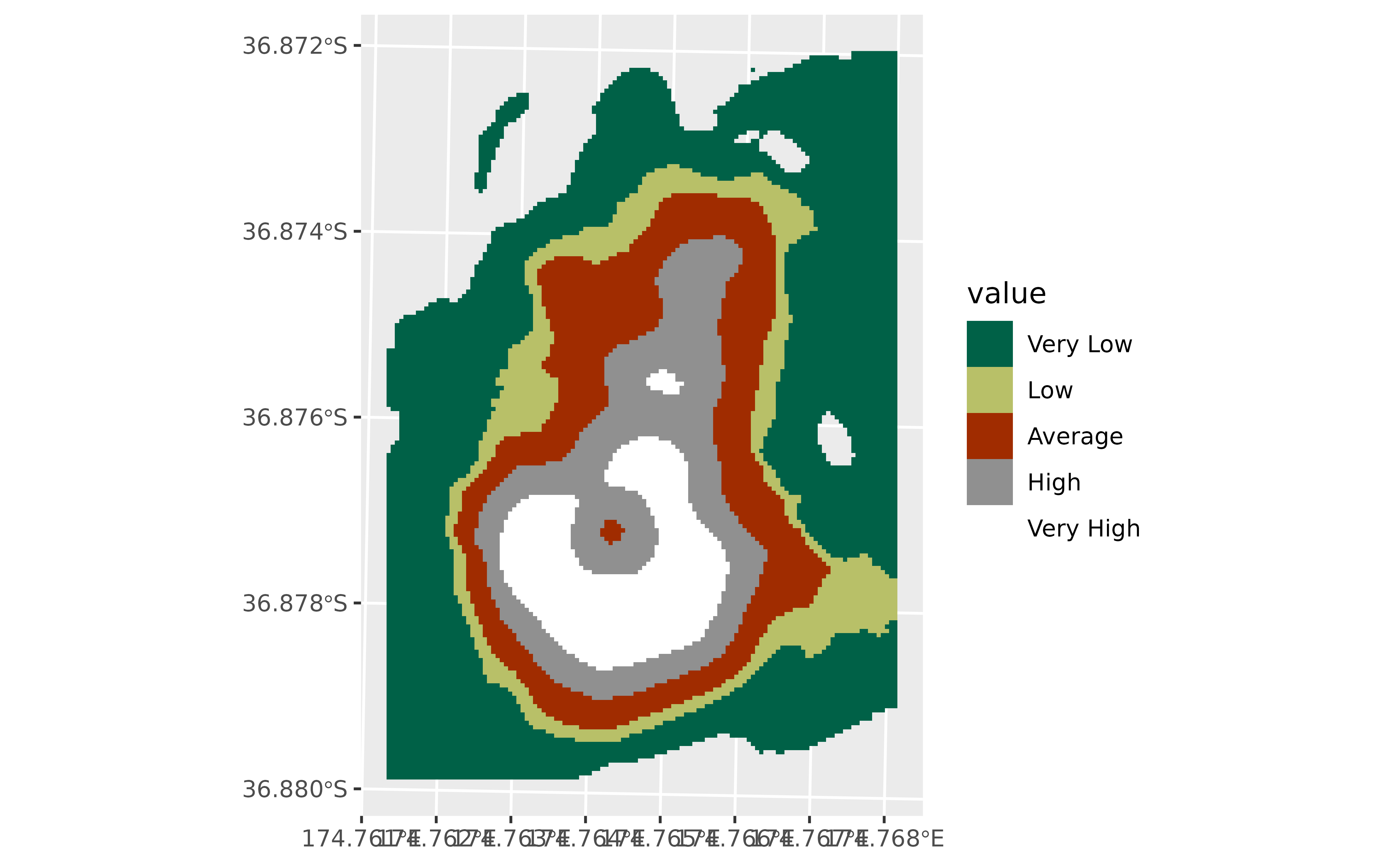# With limits and breaks
ggplot() +
geom_spatraster(data = volcano2_rast) +
scale_fill_hypso_tint_b(
breaks = seq(75, 200, 25),
palette = "wiki-2.0_hypso",
limits = my_lims
)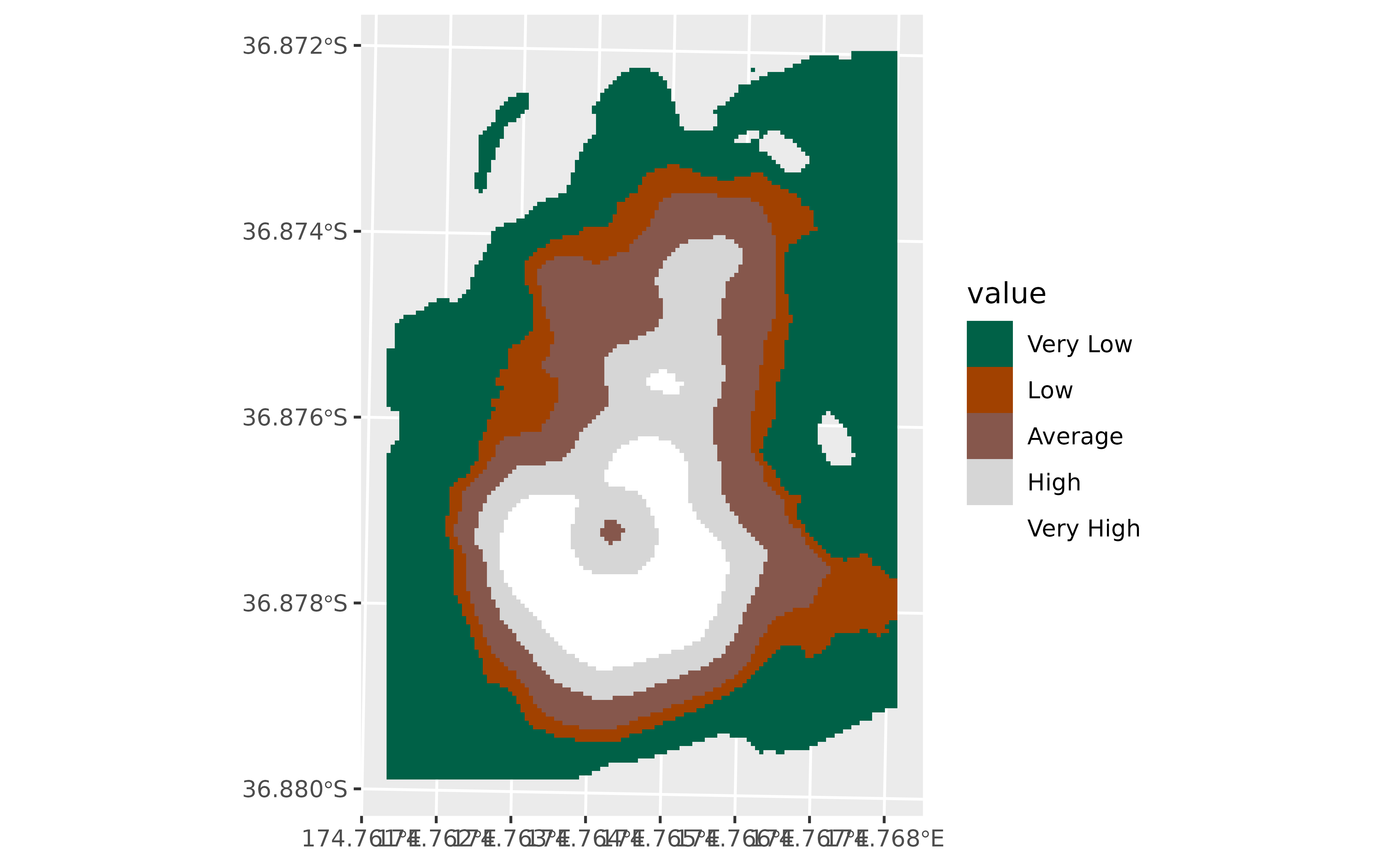# With discrete values
factor <- volcano2_rast %>% mutate(cats = cut(elevation,
breaks = c(100, 120, 130, 150, 170, 200),
labels = c(
"Very Low", "Low", "Average", "High",
"Very High"
)
))

ggplot() +
geom_spatraster(data = factor, aes(fill = cats)) +
scale_fill_hypso_d(na.value = "gray10", palette = "dem_poster")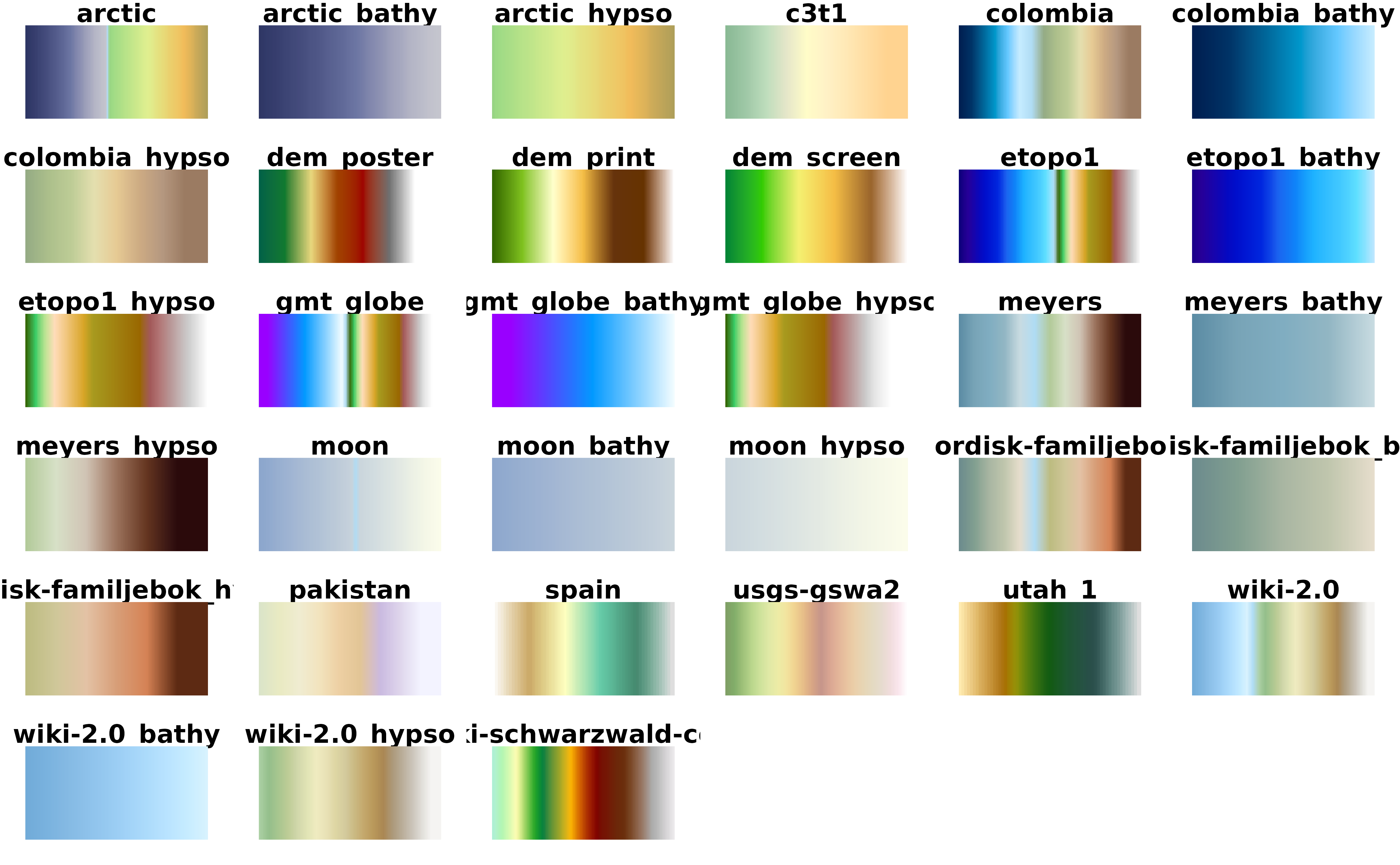# Tint version
ggplot() +
geom_spatraster(data = factor, aes(fill = cats)) +
scale_fill_hypso_tint_d(na.value = "gray10", palette = "dem_poster")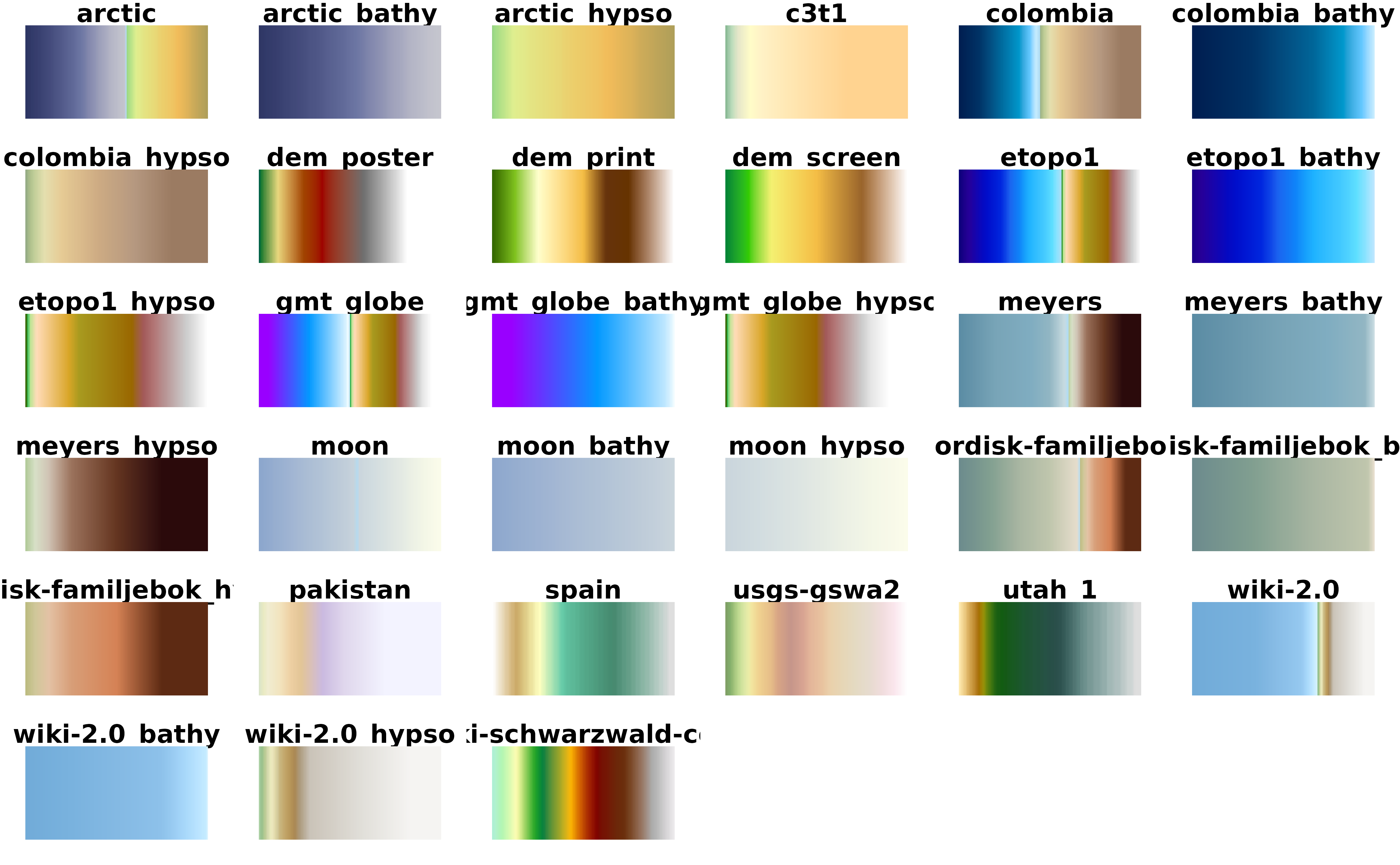# }
# Display all the cpl_city palettes

pals <- unique(hypsometric_tints_db$pal) # Helper fun for plotting ncols <- 128 rowcol <- grDevices::n2mfrow(length(pals)) opar <- par(no.readonly = TRUE) par(mfrow = rowcol, mar = rep(1, 4)) for (i in pals) { image( x = seq(1, ncols), y = 1, z = as.matrix(seq(1, ncols)), col = hypso.colors(ncols, i), main = i, ylab = "", xaxt = "n", yaxt = "n", bty = "n" ) } par(opar)# Display all the cpl_city palettes on version 2 pals <- unique(hypsometric_tints_db$pal)

# Helper fun for plotting

ncols <- 128
rowcol <- grDevices::n2mfrow(length(pals))